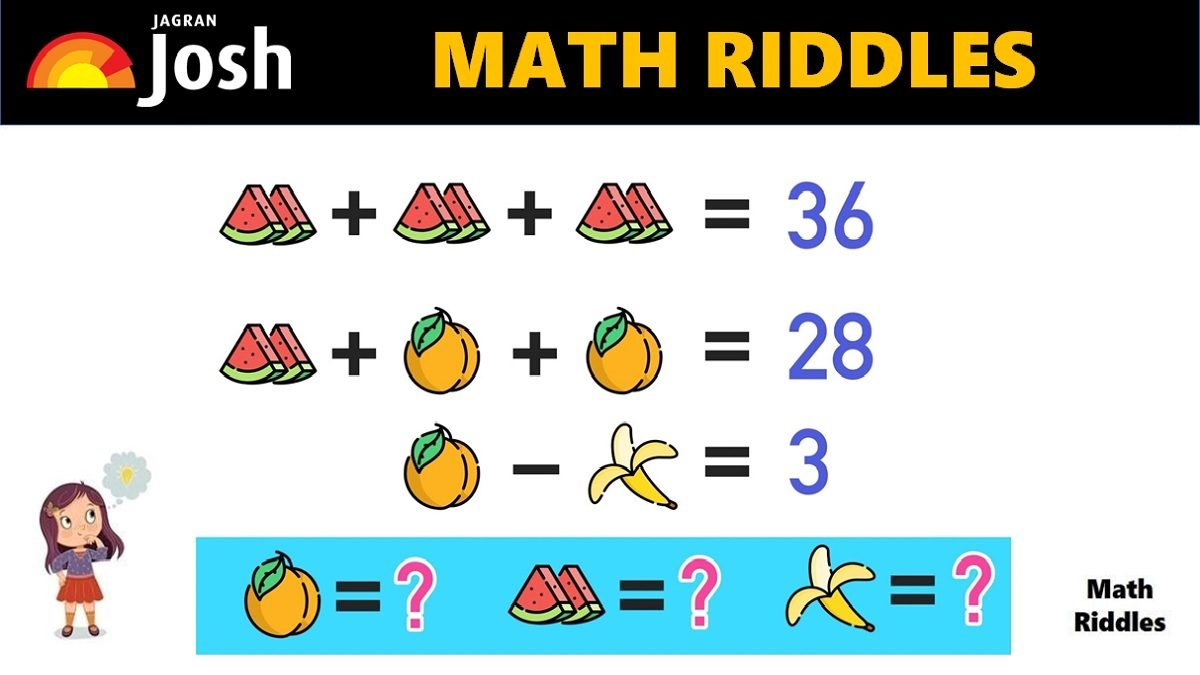# Math Riddles With Answers: Can You Find The Value of Each Fruit in 20 Seconds?

Are you a math genius? Only 1% with a high IQ can solve this tricky 'apple, banana, coconut' math picture puzzle in 20 seconds.Math Riddles With Answers: Can You Find The Value of Each Fruit in 20 Seconds?

Mathematics is a subject that involves basic arithmetic operations such as addition, subtraction, multiplication, and division. We learn these basic math skills at the school level. We learn the usage of numbers, formulas, concepts such as algebra, etc which means we can definitely solve math riddles. Here, we have math riddles for kids, math riddles for adults, math riddles for class 7, class 8, class 9, and so on. Let us check your foundations of math with these tricky math riddles.

Can you find the value of each fruit in this math riddle within 20 seconds?

## Math Riddles with Answers: Solution

Row 1: There are 3 watermelons (2 pieces each) which equals to 36.

So, we get 3 watermelons = 36, if we divide both sides (LHS and RHS) by 3 then we get the result that value of 1 watermelon (2 pieces) is equal to 12 and each single piece is 6. Now, we will use this value of 1 watermelon to solve the next row of the problem. See picture below to understand better.

Find The Hidden Object In The Picture Puzzle In 11 Seconds, Only 1% Creatives Can Solve This

Picture Puzzle: Find the Mistake In 11 Seconds, High Observational Skills Required

Row 2: Now, we have 1 watermelon + 1 orange + 1 orange = 28. We know that 1 watermelon equals 12, so we apply the value and solve the equation to find the value of 1 orange. See the picture below for better understanding.

So, now we know the value of 1 orange is 8. Let us find out the value of 1 banana.

Picture Puzzle: Only 1% Genius Can Find The Hidden Words In The Picture in 11 seconds

Row 3: Now, we got 1 orange – 1 banana = 3.

We already know that value of 1 orange is 8. So, we apply the value of 1 orange to the equation and figure out the value of 1 banana.

Great Work! 99% of people fail to find the right answer.

We found the value of each fruit. The value of 1 watermelon is 12, the value of 1 orange is 8, and the value of 1 banana is 5.

## Tell us in comments: Did you solve this math puzzle correctly in 20 seconds?

Check out more amazing tricky puzzles!

Picture Puzzle: How Sharp Is Your Vision? Find Hidden Animals In The Picture In 11 Seconds

Picture Puzzle: Find Two Same Emojis In 11 Seconds, 99% People Failed To Find Them

Picture Puzzle: Find the Mistake In 11 Seconds, High Observational Skills Required

Picture Puzzle: Genius Can Tell How Many Girls Are There In This Picture In 11 Seconds

Picture Puzzle: Test Your Intelligence Level, 1% Genius Can Spot All 6 Hidden Words in 11 Seconds

Get the latest General Knowledge and Current Affairs from all over India and world for all competitive exams.
खेलें हर किस्म के रोमांच से भरपूर गेम्स सिर्फ़ जागरण प्ले पर